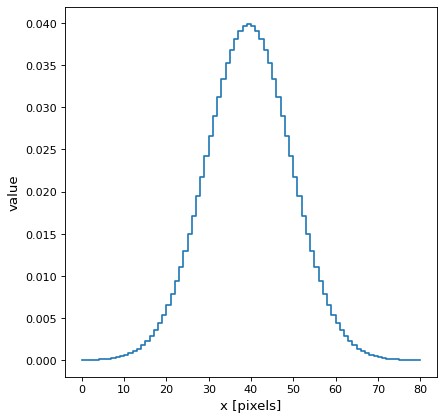# Gaussian1DKernel¶

class astropy.convolution.Gaussian1DKernel(stddev, **kwargs)[source]

1D Gaussian filter kernel.

The Gaussian filter is a filter with great smoothing properties. It is isotropic and does not produce artifacts.

Parameters
stddevnumber

Standard deviation of the Gaussian kernel.

x_sizeodd int, optional

Size of the kernel array. Default = 8 * stddev

modestr, optional
One of the following discretization modes:
• ‘center’ (default)

Discretize model by taking the value at the center of the bin.

• ‘linear_interp’

Discretize model by linearly interpolating between the values at the corners of the bin.

• ‘oversample’

Discretize model by taking the average on an oversampled grid.

• ‘integrate’

Discretize model by integrating the model over the bin. Very slow.

factornumber, optional

Factor of oversampling. Default factor = 10. If the factor is too large, evaluation can be very slow.

Examples

Kernel response:

import matplotlib.pyplot as plt
from astropy.convolution import Gaussian1DKernel
gauss_1D_kernel = Gaussian1DKernel(10)
plt.plot(gauss_1D_kernel, drawstyle='steps')
plt.xlabel('x [pixels]')
plt.ylabel('value')
plt.show()


()